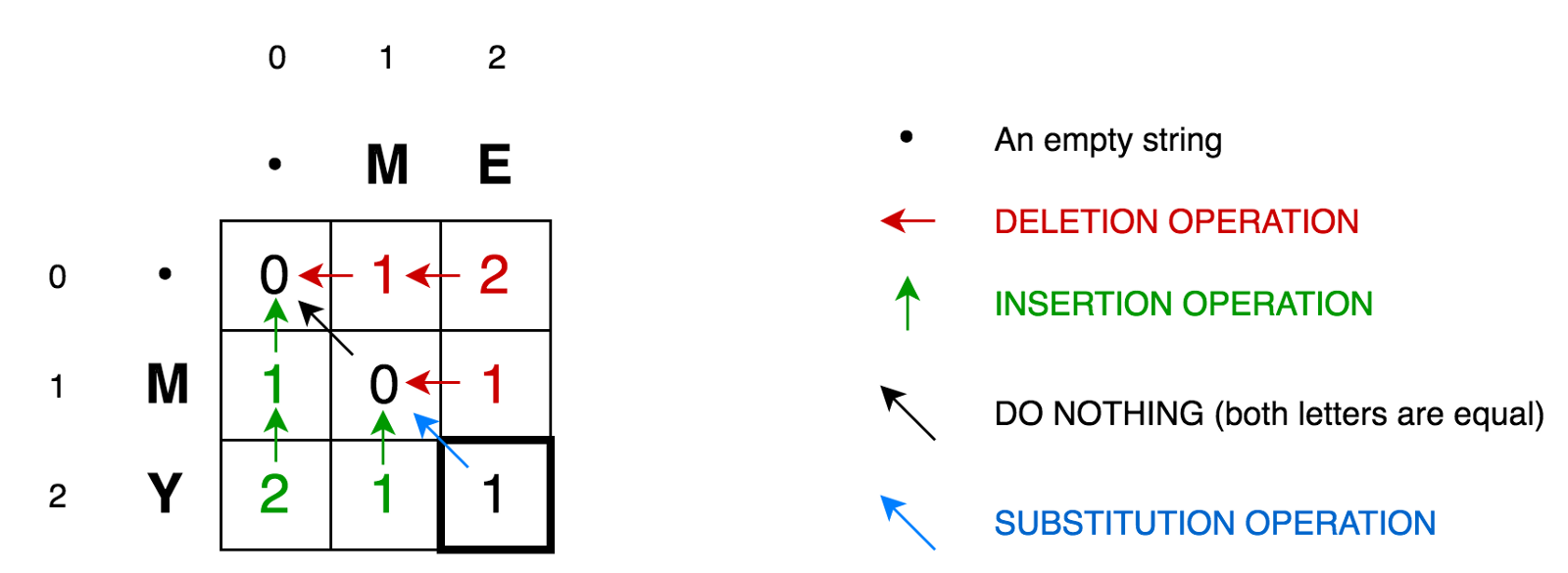Levenshtein distance calculates the number of operations needed to change one word to another by applying single-character edits (insertions, deletions or substitutions).

The reference explains this concept very well. For consistency, I extracted a paragraph from it which explains the operations in Levenshtein algorithm. The source of the following paragraph is the first reference of this article.

Levenshtein Matrix• Cell (0:1) contains red number 1. It means that we need 1 operation to transform M to an empty string. And it is by deleting M. This is why this number is red.
• Cell (0:2) contains red number 2. It means that we need 2 operations to transform ME to an empty string. And it is by deleting E and M.
• Cell (1:0) contains green number 1. It means that we need 1 operation to transform an empty string to M. And it is by inserting M. This is why this number is green.
• Cell (2:0) contains green number 2. It means that we need 2 operations to transform an empty string to MY. And it is by inserting Y and M.
• Cell (1:1) contains number 0. It means that it costs nothing to transform M into M.
• Cell (1:2) contains red number 1. It means that we need 1 operation to transform ME to M. And it is by deleting E.
• And so on…

## Examples

Characters: (( sentenceOneWords ))
Characters: (( sentenceTwoWords ))
Levenshtein Distance: (( levenshteinDistance ))

Planted: by ;

L Ma (2019). 'Levenshtein Distance', Datumorphism, 05 April. Available at: https://datumorphism.leima.is/cards/math/levenshtein-distance/.